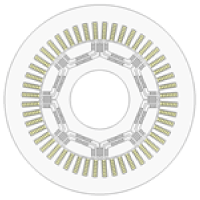## [W-OP-166] Sensitivity Study of Configuration of Large Scale Multi-Objective Optimization of a PMSM

Contents
1. Introduction
2. Optimization of a PMSM
3. Sensitivity study
3.1 Design variable range
3.2 Population size
3.3 FEA model fidelity
4. Summary

### 1. Introduction

The feasible solution space of large scale multi-objective optimization schemes is sensitive to the configuration (population size, objectives, constraints, and design variables). This report analyzes the sensitivity of the feasible solution space to the configuration parameters in the design optimization of a permanent-magnet synchronous machine. “Configuration sensitivity” is key to computational efficiency especially where multiple objectives and constraints apply, where the number of design variables is large, and where diverse technologies are required in the design process — as, for example, in automotive electrification where experienced-based design is limited.

### 2. Optimization of a PMSM

An optimization problem is defined for a 8 poles 48 slots PMSM as shown in Fig. 1. The overall objective is to find optimal current vector strategies and rotor geometries that satisfy the detailed objectives and constraints in Table 1. The number of the geometrical design variable is 20. The feasible solution area is shown in Fig. 2. In the optimization, the configuration is the maximum one which consists of widest ranges for design variables, the largest population size and the highest fidelity of FEA model as described in the following sections. The feasible solution space is quite small due to severe objectives and constraints.Fig. 1 Motor topology

Table 1 Objective functions and constraints

 Objective functions Constraints Torque Maximize >100 (Nm) (low speed) >30 (Nm) (high speed) Torque ripple rate Minimize >0.35 Loss Minimize >1,200 (W) (low speed), >3,000 (W) (high speed) Voltage Minimize >300 (V) Stress Minimize >200 (MPa)

It shows an initial motor geometry of the IPMSM to be optimized. The machine has 8 poles, 48 slots with two layers of magnets. The design variables in the optimization are 20 dimensions, and 4 for current amplitudes, and phases both at low and high speeds

Protected content here, for members only.
You need to sign in as a JMAG software regular user (paid membership) or JMAG WEB MEMBER (free membership).

By registering as a JMAG WEB MEMBER, you can browse technical materials and other member-only contents for free.
If you are not registered, click the “Create an Account” button.

Remember me